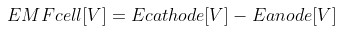Request a Tool

# Electromotive Force Calculator

EMF
0

#### Formula• Ecathode [V] = potential of the cathode (in volts)
• Eanode [V] = Eanode is the potential of the anode (in volts)

#### Defination / Uses

EMF is an abridgment of the electromotive force. By description, EMF is a force or an electric pressure which generates current in a circuit.

Find the potential of a metal electrode, one way is to check the galvanic series and find the particular metal in the table. Second way is to calculate it using the Nerst equation, also known as the cell potential equation.

Daniell cell-simple electrochemical cell invented in 1836 by John Frederic Daniell (British chemist and meteorologist). In this cell, bobby is immersed in a answer of bobby (II) sulfate and zinc is immersed in a result of zinc sulfate.

Check the metal's potential: electrode potential of zinc of is -0.76 V, and of the copper is +0.34 V ⇒ because of lower potential zinc is the anode, and copper is the cathode. Type these values into our calculator. Calculate the EMF value of the electrochemical cell using the equation:
EMFcell = +0.34 V - (-0.76 V) = 1.10 V
Here it is! The electromotive force of the Cell's schema:
(−) Zn | Zn2+ || Cu2+ | Cu (+)